Tip: Other languages are Google-Translated. You can visit the English version of this link.

## Vlookup formula – the shipping cost calculator

The VLOOKUP function can be used to calculate shipping cost based on specified weight of an item in Excel.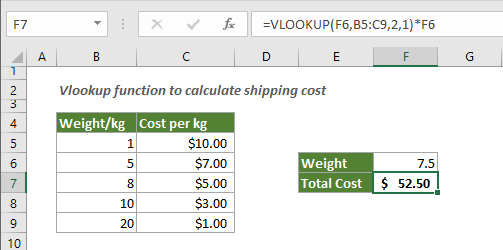#### How to calculate shipping cost based on certain weight in Excel?

Please create a table containing two lists “Weight” and “Cost” as the below sample table shown. In this case, to calculate the total shipping cost based on the specified weight in F4, please do as follows.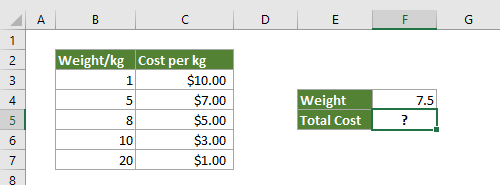Generic formula

=VLOOKUP(lookup_value,table_array,col_index,[range_lookup])*lookup_value

Arguments

• Lookup_value: The criteria value you are searching for. It must be in the first column of the table_array range.
• Table_array: The table contains two or more columns where the lookup value column and the result value column locating.
• Col_index: The specific column number (it is an integer) of the table_array, which you will return the matched value from.
• Range_lookup: This is a logical value that determines whether this VLOOKUP function will return an exact match or an approximate match.

1. Select a blank cell to output the result (here I select F5), copy the below formula into it.

=VLOOKUP(F4,B3:C7,2,1)*F4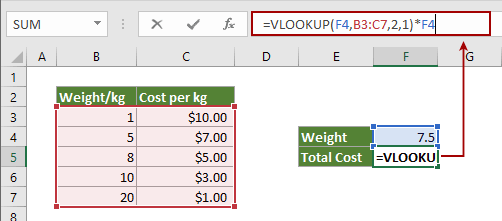2. Press the Enter key to get the total shipping cost.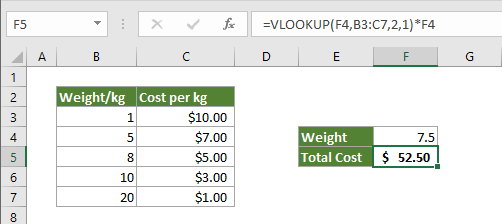Notes: in the above formula

• F4 is the cell containing the specified weight you will calculate the total shipping cost based on;
• B3:C7 is the table_array containing the weight column and the cost column;
• Number 2 represents the cost column, which you will return the matched cost from;
• Number 1 (can be replaced with TRUE) means that it will return an approximate match if an exact match cannot be found.

How this formula works

• Because of the approximate match mode, the values in the first column must be sorted in ascending order in case of returning the incorrect value.
• Here the formula =VLOOKUP(F4,B3:C7,2,1) lookups the cost for certain weight 7.5 in the first column of the table range. As 7.5 haven’t been found, it matches the next smallest weight 5 and gets its cost \$7.00. And this cost is finally multiplied by the certain weight 7.5 to get the total shipping cost.

#### How to calculate shipping cost with minimum charge in Excel?

Supposing the shipping company has a rule stipulates that the minimum shipping cost is \$7.00 no matter what the weight is. Here is the formula for you:

=MAX(VLOOKUP(F4,B3:C7,2,1)*F4,7)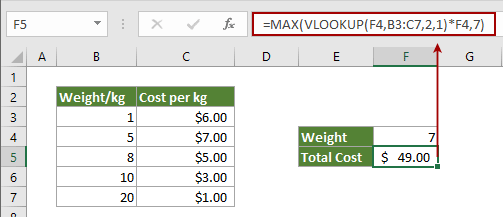Applying the above formula, the result of the total shipping cost will no less than \$7.00.

#### Related functions

The VLOOKUP function
The Excel VLOOKUP function searches for a value by matching on the first column of a table and returns the corresponding value from a certain column in the same row.

#### Related formulas

Lookup values from another worksheet or workbook
This tutorial explains how to use VLOOKUP function to search for values from another worksheet or workbook.
Click to know more...

Lookup values across multiple worksheets with dynamic sheet name
In many cases, you may need to collect data across multiple worksheets for summary. This article provides methods for you to easily lookup specific values across worksheets with dynamic sheet name.
Click to know more...

Vlookup and return matched values in multiple columns
Normally, applying the Vlookup function can only return the matched value from one column. Sometimes, you may need to extract matched values from multiple columns based on the criteria. Here is the solution for you.
Click to know more...

Vlookup to return multiple values in one cell
Normally, when applying the VLOOKUP function, if there are multiple values that match the criteria, you can only get the result of the first one. If you want to return all matched results and display them all in a single cell, how can you achieve?
Click to know more...

Vlookup and return entire row of a matched value
Normally, using the vlookup function can only return a result from a certain column in the same row. This article is going to show you how to return the whole row of data based on specific criteria.
Click to know more...Say something here...
symbols left.